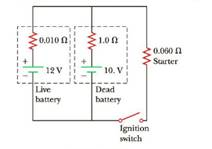Chapter 18, Problem 28P

Chapter
Section
Textbook Problem

A dead battery is charged by connecting it to the live battery of another car with jumper cables (Fig. P18.28). Determine the current in (a) the starter and in (b) the dead battery.Figure P18.28

(a)

To determine

The current in the starter.

Explanation

Given Info:

Live battery voltage is Vlive is 12V, Dead battery voltage is Vdead is 10V, Resistance R1 is 0.010Ω Resistance R2 is 1.0Ω, Resistance R3 is 0.060Ω.

The below figure shows the direction of current flow in the circuit

• Vlive is voltage in live battery
• Vdead is voltage in dead battery
• R1,R2,R3 is corresponding resistance in the circuits
• I1,I2,I3 is corresponding current in the circuits

Formula to calculate the expression for the current in the live batteries by Kirchhoff’s voltage law

VliveR1I1R3I3=0 (1)

Substitute 12V for Vlive,0.010Ω for R1,0.060Ω for R3,in the above equation to find the expression for I1

12.0V0.010ΩI10.060ΩI3=012.0V0.060ΩI3=0.010ΩI1(12.0V0.060ΩI30.010Ω)=I1I1=1200A6.0I3 (I)

Formula to calculate the expression for the current in the dead batteries by Kirchhoff’s voltage law

Substitute 10V for Vdead,1

(b)

To determine

The current in the dead battery.

Still sussing out bartleby?

Check out a sample textbook solution.

See a sample solution

The Solution to Your Study Problems

Bartleby provides explanations to thousands of textbook problems written by our experts, many with advanced degrees!

Get Started

Find more solutions based on key concepts